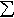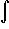#Interactive Real Analysis

Next | Previous | Glossary | Map

## 7.4. Lebesgue Integral

### Definition 7.4.3: Lebesgue Integral for Simple Function

If s(x) =an XAn(x) is a simple function and m(An) is finite for all n, then the Lebesgue Integral of s is defined ass(x) dx =an m(An)
If E is a measurable set, we defineE s(x) dx =XE(x) s(x) dx
Next | Previous | Glossary | Map